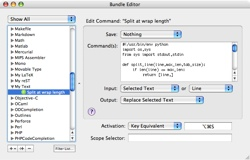# Explicitly splitting long lines in TextMate

Sometimes, when you’re just in the flow of typing text (like for a paper) you might forget about some of the personal rules you set up on how you want your textfiles formated. I, for example, prefer it, when the length of each line isn’t above 78 characters. I don’t really want this an automatic method for formating source code files, but at least for some LaTeX/reST/Markdown texts this would be nice. But while TextMate offers a small command in the “Text” menu to unwrap paragraphs, I didn’t find anything for actually splitting (and not just softwrapping) long lines.

Not really a problem so let’s write a simple command for this. And here is the result. I don’t know if it works in all cases, but I tested it for situations where nothing is selected or whole lines are selected and it seems to work there.

Warning: Don’t try this command when you don’t have full lines selected. This command wasn’t built for this and I haven’t yet found a way to check what kind of stuff got selected :) And don’t try it on program source code ;-)

```#!/usr/bin/env python
import os,sys
from sys import stdout,stdin

def split_line(line,max_len,tab_size):
if len(line) <= max_len:
return [line,]

prefix = ''
prefix_length = 0
if line in (' ',"\t"):
for c in line:
if c not in (' ',"\t"):
break
else:
prefix += c
if c == '\t':
prefix_length+=tab_size

lines = []
current_line = []
line_no = 0
words = line.split(" ")
chars = prefix_length
if len(words)==1:
return [line,]
while len(words) > 0:
if (chars+len(words)) <= max_len:
word = words.pop(0)
current_line.append(word)
chars += len(word)+1
else:
if line_no > 0:
lines.append("%s%s"%(prefix," ".join(current_line)))
else:
lines.append(" ".join(current_line))
current_line = []
line_no+=1
chars = prefix_length
if len(current_line) > 0:
if line_no > 0:
lines.append("%s%s"%(prefix," ".join(current_line)))
else:
lines.append(" ".join(current_line))
return lines

if __name__ == '__main__':
max_len = int(os.getenv('TM_WRAP_LENGTH',78))
tab_size = int(os.getenv('TM_TAB_SIZE',4))
for line in stdin:
stdout.write("\n".join(split_line(line,max_len,tab_size)))

```

And here’s the configuration for this command. I simply put it into a dummy bundle handling all my private extensions to the “Text” bundle … well, actually this is the first extensions ;-)Warning: The usual stuff. Use this on your own risk etc.

I hope this might be useful for some of you :-)